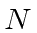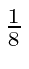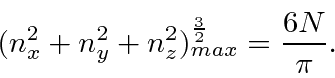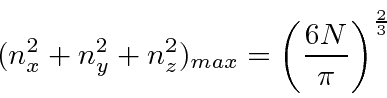## Sample Test Problems

1. * Calculate the Fermi energy forparticles of massin a 3D cubic box'' of side. Ignore spin for this problem.
The energy levels are given in terms of three quantum numbers.The number of states with inside some(of a a sphere inspace) isSo forparticles filling the levels,The energy corresponding to this is the Fermi energy.2. * We putfermions of massinto a (cold) one dimensional box of length. The particles go into the lowest energy states possible. If the Fermi energy is defined as the energy of the highest energy particle, what is the Fermi energy of this system? You may assume that there are 2 spin states for these fermions.
Jim Branson 2013-04-22